# Thermoelectrics

## The Science of Thermoelectric Materials

### The Seebeck Effect

In a thermoelectric material there are free electrons or holes which carry both charge and heat. To a first approximation, the electrons and holes in a thermoelectric semiconductor behave like a gas of charged particles. If a normal (uncharged) gas is placed in a box within a temperature gradient, where one side is cold and the other is hot, the gas molecules at the hot end will move faster than those at the cold end. The faster hot molecules will diffuse further than the cold molecules and so there will be a net build up of molecules (higher density) at the cold end. The density gradient will drive the molecules to diffuse back to the hot end. In the steady state, the effect of the density gradient will exactly counteract the effect of the temperature gradient so there is no net flow of molecules. If the molecules are charged, the buildup of charge at the cold end will also produce a repulsive electrostatic force (and therefore electric potential) to push the charges back to the hot end. The electric potential (Voltage) produced by a temperature difference is known as the Seebeck effect and the proportionality constant is called the Seebeck coefficient. If the free charges are positive (the material is p-type), positive charge will build up on the cold which will have a positive potential. Similarly, negative free charges (n-type material) will produce a negative potential at the cold end.### Thermopower Peak and Band Gap

In a semiconductor at high enough temperature electrons will have high enough energy to excite across the band gap. When that happens there will be both n-type carriers in the conduction band and p-type carriers in the valence band such that the resultant thermopower (absolute value of Seebeck coefficient) will be compensated (reduced) because the two contributions subtract. In a heavily doped semiconductor, where the dopants produce many majority carriers (could be either n-type or p-type) the thermopower will be reduced at high temperature due to the excitation of minority carriers of opposite sign. Although there are fewer minority carriers than majority carriers, they have a larger thermopower. This leads to a peak in the thermopower as a function of temperature.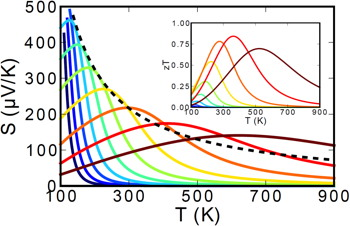The Figure above shows how the Seebeck coefficient changes when the doping changes from lightly doped (blue) to heavily doped (red).

The Temperature at which the thermopower peaks, Tmax, and the thermopower (absolute value of Seebeck coefficient) of the peak, |S|max, can be used to estimate the semiconducting band gap, Eg .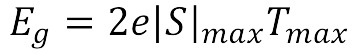This equation can be reasonably accurate when both electrons and holes have similar weighted mobility,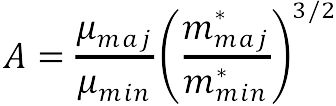(here m* is DOS mass including valley degeneracy), otherwise the carrier with higher weighted mobility will have a stronger influence and affect the estimation of band gap . The following figure can be used to estimate the error from the Band Gap equation. When the Seebeck peak is greater than 150µV/K this method for measuring Band Gap at high temperature is likely to be accurate.

### Thermoelectric Power Generation

If the hot ends of the n-type and p-type material are electrically connected, and a load connected across the cold ends, the voltage produced by the Seebeck effect will cause current to flow through the load, generating electrical power. The electrical power produced is the product of the voltage and electrical current across the load. The temperature difference provides the voltage but it is the heat flow which enables the current.

A thermoelectric generator behaves much like an ideal voltage soure with an internal resistance due largely to the resistance of the thermoelectric materials themselves. The voltage at the load is reduced from the open circuit voltage by the Ohm's law (V = IR) voltage drop due to this internal resistance. Maximum efficiency is reached when the load and internal resistances are nearly equal because this is close to the maximum power achieved from load matching.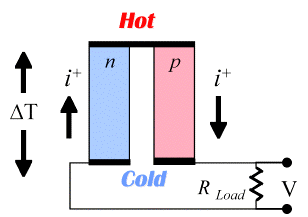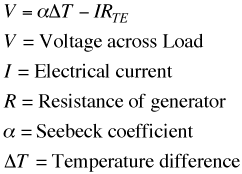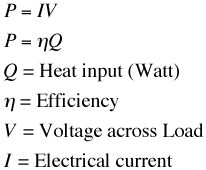The resistance of the thermoelectric elements depend on the electrical resistivity as well as the length and cross sectional area.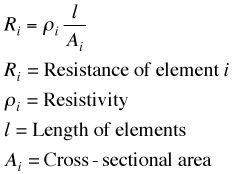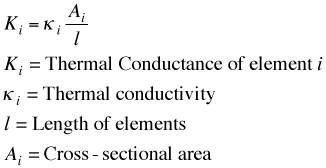Just as the power in a resistor is V2/R the power produced in a thermoelectric generator depends on the square of the voltage (Seebeck coefficient and temperature difference) divided by the resistivity. Notice also that the power per area can be arbitrarily adjusted with l (length).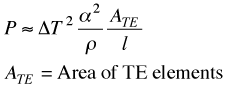The efficiency of a generator depends not just on the power produced but also how much heat is provided at the hot end. The heat input is needed for the thermoelectric process (Peltier effect) as well as normal thermal conduction (Fourier's law) and is offset by the Joule heating in the device. The Fourier's law thermal conduction of the thermoelectric materials add a thermal path from hot to cold that consumes some heat and reduces the efficiency.

It can be shown that the maximum efficiency of a thermoelectric material depends on two terms. The first is the Carnot efficiency, for all heat engines can not exceed Carnot efficiency. The second is a term that depends on the thermoelectric properties, Seebeck coefficient, electrical resistivity and thermal conductivity. These material properties all appear together and thus form a new material property which we call zT, the Thermoelectric Figure of Merit. For small temperature difference this efficiency is given by: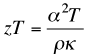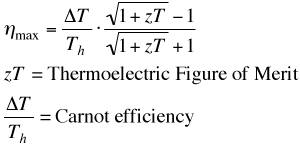Notice also that the extensive geometric parameters, length and area have dropped out of this expression for maximum efficiency. In a real generator with large temperature difference other methods and approximations are frequently used to calculate performance (see Engineering page).

Another material property that becomes important when different materials or large temperature differences are used, is the thermoelectric compatibility factor s. For power generation, the compatibility factor (see Engineering page) should not change by more than a factor of two from the hot to the cold end of a thermoelectric element.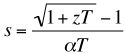##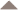### Peltier Cooling

If instead of having the heat flow drive the charge flow, we use an external electric potential to drive the heat carrying charges, then we can force heat to flow from one end to the other. The coefficient of performance and the maximum temperature drop that can be achieved is again related to the efficiency of the thermoelectric materials through the thermoelectric figure of merit zT.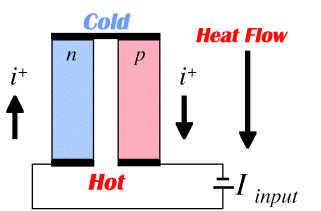Thermoelectric Materials Development A material with a large thermoelectric power factor and therefore zT, needs to have a large Seebeck coefficient (found in low carrier concentration semiconductors or insulators) and a large electrical conductivity (found in high carrier concentration metals). The thermoelectric power factor maximizes somewhere between a metal and semiconductors. Good thermoelectric materials are typically heavily doped semiconductors with carrier concentration of 1019 to 1021 carriers/cm3. To ensure that the net Seebeck effect is large, there should only be a single type of carrier. Mixed n-type and p-type conduction will lead to opposing Seebeck effect and low thermopower (defined here as absolute value of Seebeck coefficient).By having a band gap large enough, n-type and p-type carriers can be separated, and doping will produce only a single carrier type. Thus good thermoelectric materials have band gaps large enough to have only a single carrier type but small enough to sufficiently high doping and high mobility (which leads to high electrical conductivity).

A good thermoelectric material also needs to have low thermal conductivity. Thermal conductivity in such materials comes from two sources of heat transport. Phonons travelling through the crystal lattice transport heat and lead to lattice thermal conductivity. The electrons (or holes) also transport heat and lead the electronic thermal conductivity. The electronic term is related to the electrical conductivity through the Wiedeman-Franz law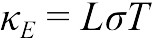where L is the Lorenz factor L. A good estimate for L (at any temperature) based only on the measured thermopower (absolute value of the Seebeck coefficient) is: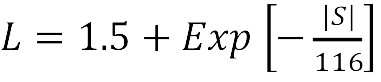where L is measured in 10-8 WΩ K-2 and S in µV/K .

Thus the greatest opportunity to enhance zT is to minimize the lattice thermal conductivity. This can be done by increasing the phonon scattering by introducing heavy atoms, disorder, large unit cells, clusters and rattling atoms.

Examples of structures with complex anions and rattling atoms are shown below in the Skutterudite and Yb14MnSb11 Phases that cant be understood with Zintl electron counting rules.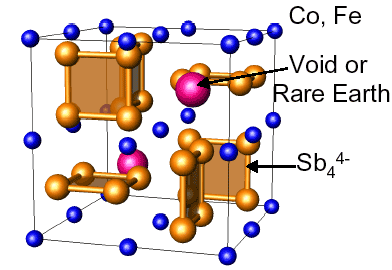Crystal Structure of Skutterudite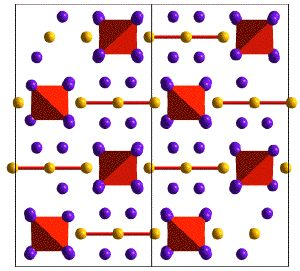Crystal Structure of Yb14MnSb11

The ideal thermoelectric material is then one which is a "Electron Crystal - Phonon Glass"  where high mobility electrons are free to transport charge and heat but the phonons are disrupted at the atomic scale from transporting heat.Thermal conductivity decrease in Skutterudites by the
introduction of various scattering mechanisms

Using these principles, a variety of high zT materials have been developed. Many materials have an upper temperature limit of operation, above which the material is unstable. Thus no single material is best for all temperature ranges, so different materials should be selected for different applications based on the temperature of operation. This leads to the use of a segmented thermoelectric generator.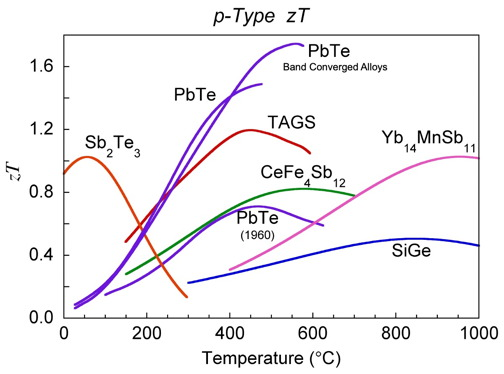zT for p-type thermoelectric materials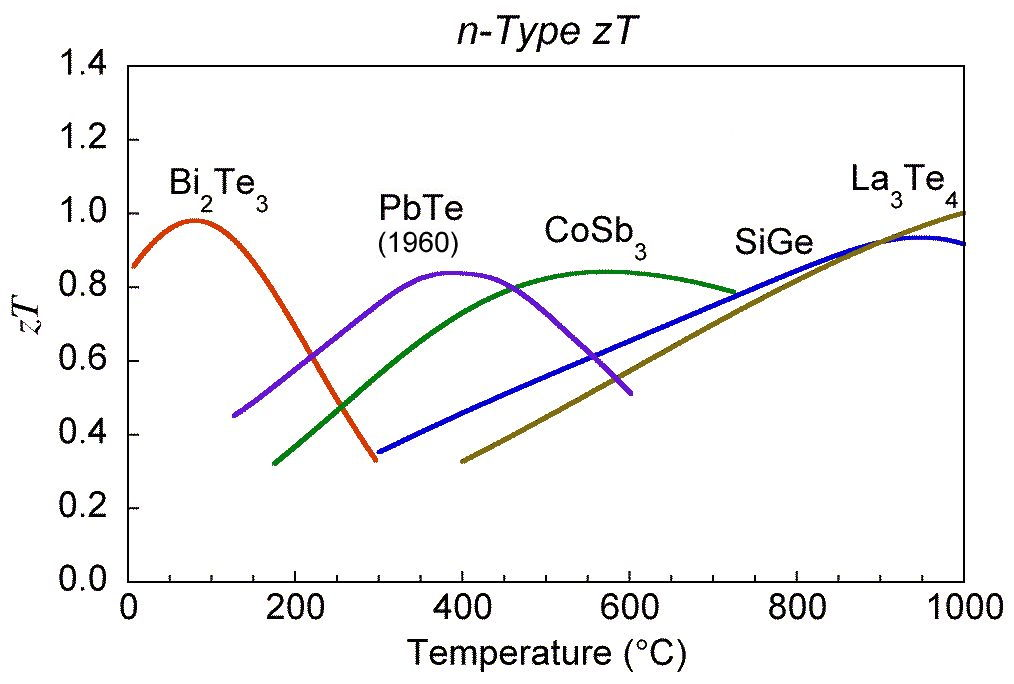zT for n-type thermoelectric materials

G. Jeffrey Snyder and Eric S. Toberer "Complex Thermoelectric Materials" Nature Materials 7, 105-114 (2008).

 CRC Handbook of Thermoelectrics, Introduction, Edited by D.M. Rowe, CRC Press, 1995.

 G. Jeffrey Snyder, Tristan Ursell. "Thermoelectric efficiency and compatibility" Physical Review Letters, Vol 91 p. 148301 (2003)

 Zachary M. Gibbs , Hyun-Sik Kim , Heng Wang , and G. Jeffrey Snyder “Band gap estimation from temperature dependent Seebeck measurement—Deviations from the 2e|S|maxTmax relation” Applied Physics Letters 106, 022112 (2015)

 Hyun Sik Kim, Zachary Gibbs, Yinglu Tang, Heng Wang, and G. J. Snyder “Characterization of Lorenz number with Seebeck coefficient measurement” APL Materials 3 041506 (2015)